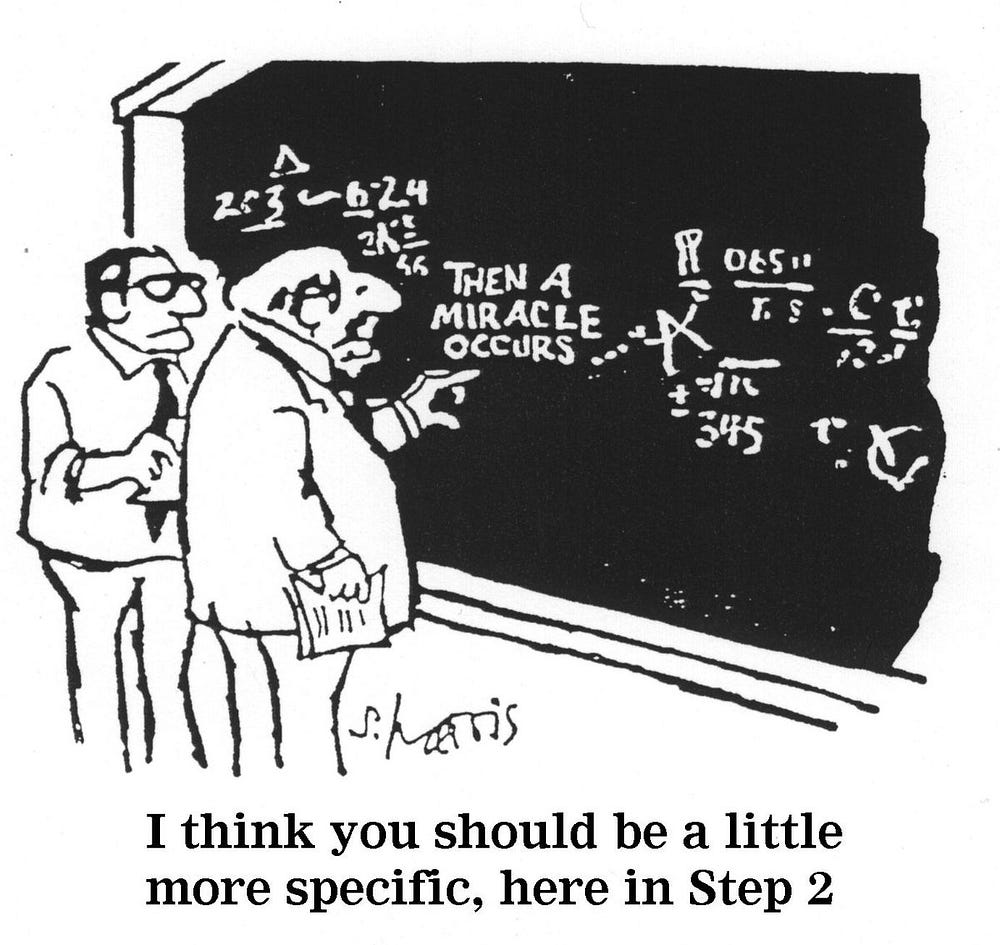# Demystifying Convolutional Neural Networks using ScoreCam

Source: Deep Learning on Medium# Demystifying Convolutional Neural Networks using ScoreCam

The Score-Cam, unlike the famous Grad-Cam, didn’t make use of gradients because the researcher believes that the propagated gradients are pretty unstable and produce random noise in gradient-based saliency maps. The unstable nature of the gradients is shown in Figure 3 where the gradient changes sharply when the input image is changed a little even though the change is not perceived by the human eye and does not change the prediction result. That’s why it is reasonable to doubt the effectiveness of gradient-based methods to reduce redundant noise.

# Methodology:

Various previous works like the CAM, GradCam etc have asserted the fact that deeper layers in CNNs capture higher-level visual information. Furthermore, convolutional features naturally retain spatial information that is lost in fully connected layers, so it is common to expect the last convolutional layer to offer the best compromise between high-level semantics and detailed spatial information, and the neurons in these layers look for class-specific semantic information in the input image. Hence in Score-Cam, we use the last layer to get the activation maps containing the balanced representations.

In contrast to GradCam and GradCam++ which use the gradient information flowing through the last layer of the CNN to represent the importance of each activation map. In Score-Cam, we use the weights of the score obtained for a specific target class. Hence, Score-Cam can get rid of the dependence on the gradient and works as a more general framework as it only requires access to the class activation map and output score of the model.

The Pipeline of Score-Cam is shown in Figure 4 and shows all the steps involved to reach the final Saliency Map.

In TotTrainain the class discriminative Saliency map of Score-Cam, the process is divided into the following steps:

1. The first step involved is passing images to a CNN model and performing a forward_pass. After the forward pass, the activations are extracted from last convolutional layer in the network.
2. Each Activation Map obtained from the last layer having shape 1xmxn is then upsampled using bilinear-interpolation to the same size as the Input Image.
3. After Upsampling the activation maps, the resultant activation maps are normalized with each pixel within the range of [0,1] to maintain the relative intensities between the pixels. The Normalization is achieved using the following formula shown in Figure 5.

4. After the Normalization of the Activation Maps is complete, the highlighted areas of the activation maps are projected on the input space by multiplying each normalized activation map(1 x W x H) with the Original Input Image(3 x W x H) to obtain a masked image M with shape 3 x W x H.

5. The Masked Images M thus obtained are then passed to Convolutional Neural Network with SoftMax output.

6. After getting the scores for each class we extract the score of the target class to represent the importance of the kth activation map.

7. Then we compute the sum across all the activation maps for the linear combination between target class score and each activation map. This results in a single activation map having the same size as that of the input class.

8. Finally, we apply pixel-wise ReLU to the final activation map. We apply ReLU because we are interested only in the features that have a positive influence on the class of interest.

# IMPLEMENTATION OF SCORE CAM IN KERAS:

We will follow the same pipeline described early both in the figure and methodology.

1. We use VGG16 pre-trained on Imagenet as our model for the whole pipeline.